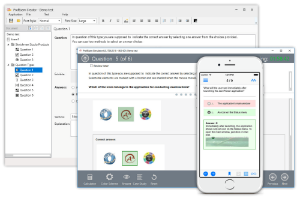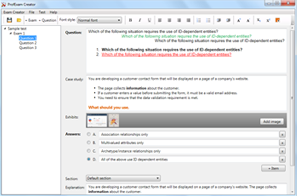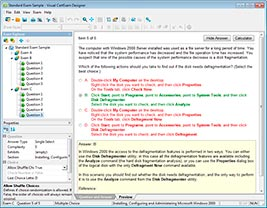## File Info

 Exam Introduction to Programming Using Java Number 98-388 File Name Introduction to Programming Using Java.actualtests.98-388.2019-11-12.1e.6q.vcex Size 119 Kb Posted November 12, 2019 Downloads 65

### How to open VCEX & EXAM Files?

Files with VCEX & EXAM extensions can be opened by ProfExam Simulator.

Discount: 20%

## Demo Questions

Question 1
You have the following code segment. Line numbers are included for reference only.What is the output of line 07?

• A: -44
• B: -40.0
• C: 40.0
• D: 44.0

Question 2
You need to analyze the following code segment. Line numbers are included for reference only.What is the output of line 12 when you run printInt()?

• A: 0
• B: 1
• C: 2
• D: 3

Question 3
The question requires that you evaluate the underlined text to determine if it is correct.
You should use an int data type to store the numeric value 3,000,000,000 (3 billion) so that the least amount of memory is used.
Review the underlined text. If it makes the statement correct, select “No change is needed.” If the statement is incorrect, select the answer choice that makes the statement correct.

• A: No change is needed.
• B: a short
• C: a byte
• D: a long

Question 4
You need to evaluate the following code segment:What happens when the code segment is run?

• A: iNum has a value of 0.
• B: An exception is thrown.
• C: iNum has a value of 2.
• D: iNum has a value of 3.

Question 5
You work as a Java programmer.
You need to convert a numeric String to a primitive double value.
What code segment should you use?
Double.valueOf(numberString);

• A: double.parseDouble(numberString);
• B: String.parseDouble(numberString);
• C: Double.parseDouble(numberString);
• D:

CONNECT US##### PROFEXAM WITH A 20% DISCOUNT

You can buy ProfExam with a 20% discount..

HOW TO OPEN VCEX AND EXAM FILES

Use ProfExam Simulator to open VCEX and EXAM filesHOW TO OPEN VCE FILES

Use VCE Exam Simulator to open VCE files